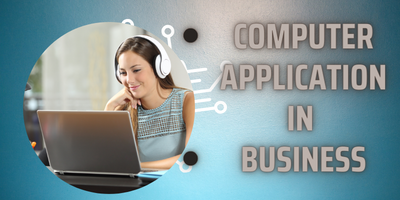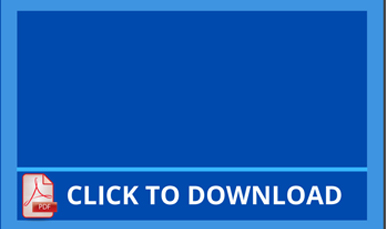Computer Application III (Eng.)

Our BrochuresComputer Application III (Eng.)

Computer Application in Business
(Chapter-wise)
IMPORTANT QUESTION
Computer Application in Business, Exam Related Important Question with BEST PDF Solution and by Video's.  here you can easily learn by Download all Important PDF Related B.Com 3rd Year Examination . Student who have Knowledge about Computer easily done Examination 100% by Watching these PDF. and who have no idea and not much knowledge or Part time job Student easily learn by watching Simple video's and Downloading PDF Files attached in this Area.

ALL THE BEST FOR EXAM

For Download PDF 3rd Year (Computer Application in Business) Student's have to pay Rs.199 only for Computer Application Notes.(Computer (E) + Economics (E) @ 299 Rs)

Pay by Debit/credit Card/Net Banking  Or Paytm (Secure)

Find Available

OUR PDF DocumentsChapter -1 (Basic Concept)

Chapter -1 (Basic Concept)Computer Application - Syllabus

Computer Application - SyllabusSample Paper - 1

Sample Paper - 1Sample Paper - 2

Sample Paper - 2Sample Paper - 3

Sample Paper - 3Chapter -2 (Component of Computer)

Chapter -2 (Component of Computer)Chapter -4 (Word Processing)

Chapter -4 (Word Processing)Chapter -5-6 (Spreadsheet Application)

Chapter -5-6 (Spreadsheet Application)Chapter -7 (Presentation Software)

Chapter -7 (Presentation Software)Chapter -3 (Introduction of Internet)

Chapter -3 (Introduction of Internet)Practical -1 (Marksheet Formula)

Practical -1 (Marksheet Formula)Practical -2 (Payroll Formula)

Practical -2 (Payroll Formula)Practical -3 (Loan Formula)

Practical -3 (Loan Formula)Practical -4 (Ratio Analysis Formula)

Practical -4 (Ratio Analysis Formula)Practical -5 (Mix Formula-1)

Practical -5 (Mix Formula-1)Practical -6 (Mix Formula-2)

Practical -6 (Mix Formula-2)SOL - Non College Previous Paper

Find Available